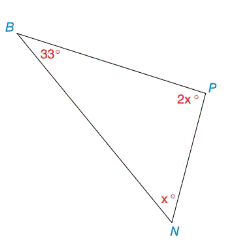Chapter 2.4, Problem 37E### Elementary Geometry for College St...

6th Edition
Daniel C. Alexander + 1 other
ISBN: 9781285195698

#### Solutions

Chapter
Section### Elementary Geometry for College St...

6th Edition
Daniel C. Alexander + 1 other
ISBN: 9781285195698
Textbook Problem
1 views

# On a map, three Los Angeles suburbs are located at points N (Newport Beach), P (Pomona), and B (Burbank). With angle measures as indicted, determine m ∠ N and m ∠ P .To determine

To find:

The value of mN and mP.

Explanation

Given:

Figure (1)

Approach:

The sum of interior angles of a triangle is equal to 180°.

Calculation:

mB+mP+mN=180°

Substitute 33° for mB, x for mN and 2x for mP in equation (1).

33°+x+2x=180°3x=180°33x

### Still sussing out bartleby?

Check out a sample textbook solution.

See a sample solution

#### The Solution to Your Study Problems

Bartleby provides explanations to thousands of textbook problems written by our experts, many with advanced degrees!

Get Started

#### let f(x) = x3 + 5, g(x) = x2 2, and h(x) = 2x + 4. Find the rule for each function. 8. fgh

Applied Calculus for the Managerial, Life, and Social Sciences: A Brief Approach

#### Use left to right elimination to solve.

Mathematical Applications for the Management, Life, and Social Sciences

Elementary Technical Mathematics

#### Convert the following percents to decimals. 121%

Contemporary Mathematics for Business & Consumers

#### The normal plane to at t = 1 has equation:

Study Guide for Stewart's Multivariable Calculus, 8th

#### An integral for the solid obtained by rotating the region at the right about the y-axis is:

Study Guide for Stewart's Single Variable Calculus: Early Transcendentals, 8th